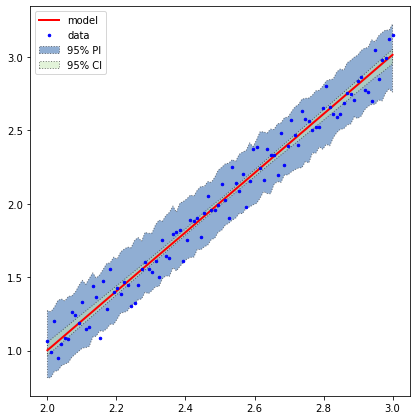# Ctypes Example¶

Author(s): Paul Miles, Joel Kulesza | Date Created: June 28, 2018

Note, this tutorial is useful for illustrative purposes. However, compiling the C++ code in Binder is non-trivial, so this is recommended as a Read-Only example.

In this example, we demonstrate how to use a model written in C++ within the context of using pymcmcstat. In this case we consider a linear model.

$$y(x;q) = m x + b + \epsilon, \quad \epsilon\sim N(0,\sigma^2), \quad q = [m, b]$$

but note that the model can be arbitrarily complex. Similar work can be performed with Fortran using the iso_c_binding module.

In :
import numpy as np
from pymcmcstat.MCMC import MCMC
import matplotlib.pyplot as plt
import ctypes
from numpy.ctypeslib import ndpointer
import pymcmcstat
print(pymcmcstat.__version__)
np.seterr(over='ignore')

1.9.0

Out:
{'divide': 'warn', 'over': 'warn', 'under': 'ignore', 'invalid': 'warn'}

# Define data set¶

To define our data set, we generate a set of points along the line, $y = 2x - 3$, and add some random noise to the response. We have plotted the data with and without the random noise added to it.

In :
# Define data set
nds = 100
x = np.linspace(2, 3, num=nds)
x = x.reshape(nds, 1)
m = 2 # slope
b = -3 # offset
noise = 0.1*np.random.standard_normal(x.shape)
y = m*x + b + noise

# plot data
plt.figure(dpi=100, figsize=(4, 4))
plt.plot(x, y, '.k', label='With noise');
plt.plot(x, m*x + b, '-r', label='Without noise');
plt.legend();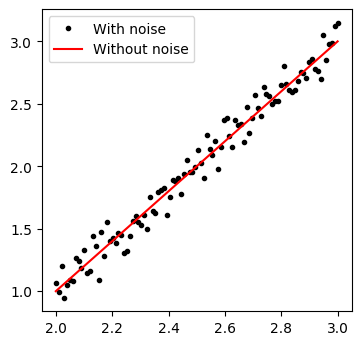# Define C++ Model¶

We define our C++ model in the file linear_model.cpp.

#include <cmath>
#include <iostream>

extern "C" {
double* linear_model(float slope, float offset, double *x, int nx) {
double* y = new double[nx];
for (int ii = 0; ii < nx; ii++) {
y[ii] = slope*x[ii] + offset;
}
return y;
}
}


You must compile the code in your terminal in order to generate an object to be called within Python. In this case, we output the compiled program to linear_model.so.

g++ -fPIC -shared -o linear_model.so linear_model.cpp


Note that .so is a common Linux convention for "shared object". On macOS this might be referred to as a dynamic library (.dylib) and on Windows this is usually called a dynamic-link library (.dll). Such objects can be inspected with the nm command on the macOS or Linux command line.

Within our Python script, we now utilize the ctypes package to make the C++ program callable within our routine. The compiled library is loaded, and the linear_model is assigned to the variable cpplm. The restype tells Python what type of variable to expect from the C++ code, and the artypes tells Python what data types to send to the C++ code. For more details, see the ctypes documentation: https://docs.python.org/3/library/ctypes.html

In :
lib = ctypes.cdll.LoadLibrary('resources/linear_model.so')
cpp_linear_model = lib.linear_model
cpp_linear_model.restype = ndpointer(dtype = ctypes.c_double, shape=(nds,))
cpp_linear_model.argtypes = [ctypes.c_float, ctypes.c_float, ndpointer(ctypes.c_double), ctypes.c_int]


# Define Python Model¶

In :
def py_linear_model(q, x):
m, b = q
return m*x + b


# Compare Model Outputs¶

In :
ycpptest = cpp_linear_model(3, 2, x, nds)
ypytest = py_linear_model([3, 2], x)
import matplotlib.pyplot as plt
fig, ax = plt.subplots()
ax.plot(x, ycpptest, ':', label='C++')
ax.plot(x, ypytest, '--', label='Python')
tmp = ax.legend()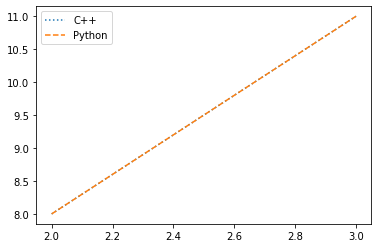Both models output the same thing!

# Define Sum-of-Squares Function¶

At this point we can define our sum-of-squares function and access the C++ code for model evaluation. We setup our evaluation such that we can easily compare a Python and C++ implementation. Note, the model type is inputted to the function by including it in the user_defined_object of the data structure.

In :
def test_ssfun(theta, data):
xdata = data.xdata
ydata = data.ydata
model_type = data.user_defined_object
nx = len(xdata)
if model_type == 'cpp':
# eval model c++ model
ymodel = cpp_linear_model(theta, theta, xdata, nx).reshape(ydata.shape)
else:
ymodel = py_linear_model(theta, xdata).reshape(ydata.shape)
# calc sos
ss = ((ymodel - ydata)**2).sum()
return ss


# Setup MCMC Simulation¶

We can now setup our MCMC simulation as we would for any other problem. Note, the C++ model has been added to the data structure.

In :
model_types = ['py', 'cpp']
results = {}
for model_type in model_types:
print('Running MCMC using model type: {}'.format(model_type))
# Initialize MCMC object
mcstat = MCMC()
# initialize parameter array
name='m',
theta0=1.7,
minimum=-10,
maximum=10)
name='b',
theta0=2.5,
minimum=-10,
maximum=100)
# update simulation options
mcstat.simulation_options.define_simulation_options(
nsimu=int(10.0e3),
method='dram',
verbosity=1,
waitbar=True)
# update model settings
mcstat.model_settings.define_model_settings(sos_function=test_ssfun)
# Run mcmcrun
mcstat.run_simulation()
results[model_type] = mcstat.simulation_results.results.copy()
print('\n')

Running MCMC using model type: py

Sampling these parameters:
name      start [      min,       max] N(       mu,   sigma^2)
m:      1.70 [   -10.00,     10.00] N( 0.00e+00,      inf)
b:      2.50 [   -10.00,    100.00] N( 0.00e+00,      inf)
[-----------------100%-----------------] 10000 of 10000 complete in 2.4 sec

Running MCMC using model type: cpp

Sampling these parameters:
name      start [      min,       max] N(       mu,   sigma^2)
m:      1.70 [   -10.00,     10.00] N( 0.00e+00,      inf)
b:      2.50 [   -10.00,    100.00] N( 0.00e+00,      inf)
[-----------------100%-----------------] 10000 of 10000 complete in 3.1 sec



The Python model runs slightly faster, but we are dealing with a very simple model. For many problems, the existing models in C++ will provide the optimal approach.

# Extract Results and Display Chain Statistics/Plots¶

We take the results of the MCMC simulation, remove the first half of the chain to account for burnin, and perform our analysis on the remaining part of the chain.

Plots:

• Marginal posterior densities
• Sampling chains
• Pairwise correlation
In :
for model_type in model_types:
print(30*'*')
print('Results using model type: {}'.format(model_type))
# Extract results
result = results[model_type]
chain = result['chain']
s2chain = result['s2chain']
sschain = result['sschain']
names = result['names']
# define burnin
burnin = int(result['nsimu']/2)
# display chain statistics
mcstat.chainstats(chain[burnin:,:], result)

******************************
Results using model type: py

------------------------------
name      :       mean        std     MC_err        tau     geweke
m         :     2.0137     0.0335     0.0023    23.2615     0.9888
b         :    -3.0263     0.0844     0.0057    22.9083     0.9822
------------------------------
==============================
Acceptance rate information
---------------
Results dictionary:
Stage 1: 0.69%
Stage 2: 5.74%
Net    : 6.43% -> 643/10000
---------------
Chain provided:
Net    : 7.46% -> 373/5000
---------------
Note, the net acceptance rate from the results dictionary
may be different if you only provided a subset of the chain,
e.g., removed the first part for burnin-in.
------------------------------
******************************
Results using model type: cpp

------------------------------
name      :       mean        std     MC_err        tau     geweke
m         :     2.0128     0.0320     0.0021    25.2899     0.9993
b         :    -3.0253     0.0805     0.0052    24.5392     0.9982
------------------------------
==============================
Acceptance rate information
---------------
Results dictionary:
Stage 1: 0.89%
Stage 2: 7.17%
Net    : 8.06% -> 806/10000
---------------
Chain provided:
Net    : 9.88% -> 494/5000
---------------
Note, the net acceptance rate from the results dictionary
may be different if you only provided a subset of the chain,
e.g., removed the first part for burnin-in.
------------------------------


Both approaches yield similar statistics and acceptance rates.

In :
from pymcmcstat import mcmcplot as mcp
# generate mcmc plots
settings = dict(
fig=dict(figsize=(4,4)))
for model_type in model_types:
print(30*'*')
print('Results using model type: {}'.format(model_type))
# Extract results
result = results[model_type]
chain = result['chain']
s2chain = result['s2chain']
sschain = result['sschain']
names = result['names']
# define burnin
burnin = int(result['nsimu']/2)
# plot density panel
f = mcp.plot_density_panel(chain[burnin:, :], names,
settings=settings)
ax = f.axes
ax.set_title(model_type)
# plot chain panel
f= mcp.plot_chain_panel(chain[burnin:, :], names,
settings=settings)
ax = f.axes
ax.set_title(model_type)
# plot pairwise correlation panel
f = mcp.plot_pairwise_correlation_panel(chain[burnin:, :], names,
settings=settings)
ax = f.axes
ax.set_title(model_type)

******************************
Results using model type: py
******************************
Results using model type: cpp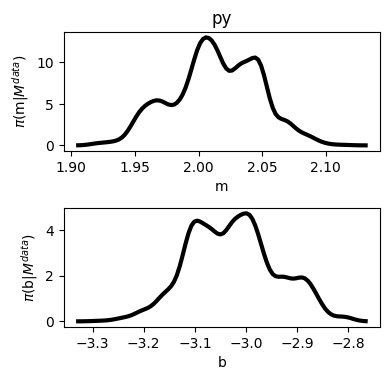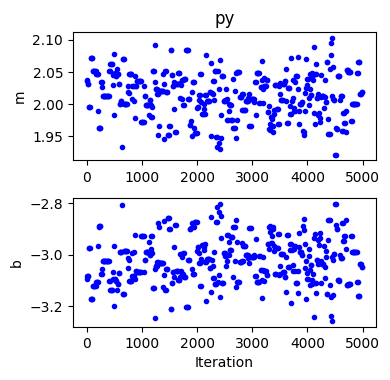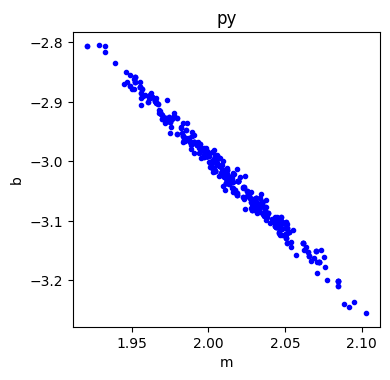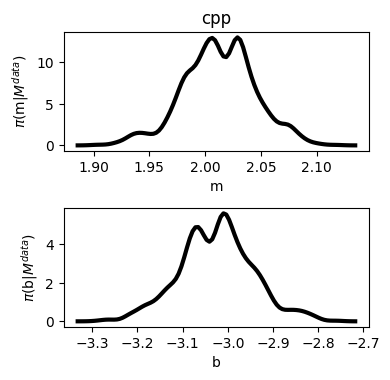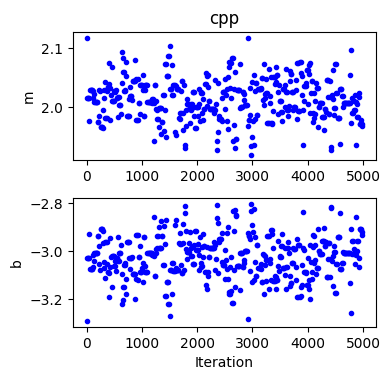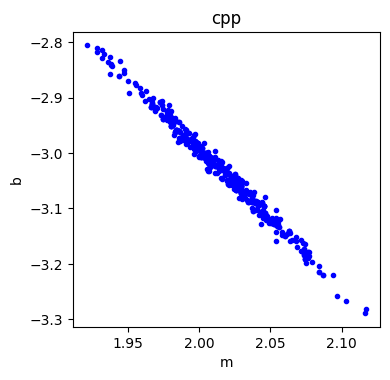# Generate/Plot Prediction Intervals¶

The C++ model can also be used in generating credible/prediction intervals.

In :
# generate prediction intervals
def pred_modelfun(preddata, theta):
return cpplm(theta, theta, preddata.xdata, nds).reshape(nds,)
mcstat.PI.setup_prediction_interval_calculation(
results=results['cpp'],
data=mcstat.data,
modelfunction=pred_modelfun)
mcstat.PI.generate_prediction_intervals()

Generating credible/prediction intervals: